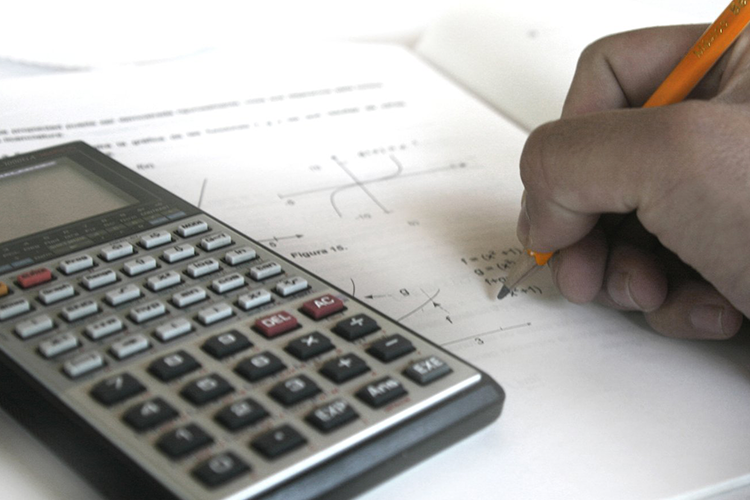Kanata Art center
HOME > MATHEMATICS > Algebra

#Course Description

The objective of this course is to prepare students for success in their Grade 11 and Grade 12 math and science courses. We will focus on introducing the fundamental concepts in a rigorous and systematic way.

### Term One

• abstraction and definitions and mathematical terms
• notation and introduction to sets
• systems of linear equations
• polynomial factoring I
• polynomial factoring II

### Term Two

• review of term one material
• systems of linear and quadratic equations
• application of linear and quadratic equations
• parametric equations
• fundamental theorem of algebra
• Vieta’s Theorem and applications
• course review

Appropriate for: Grade 7 to 10